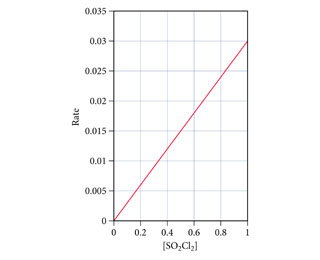# Problem: This plot shows the rate of the decomposition of SO2Cl2 into SO2 and Cl2 as a function of the concentration of SO2Cl2.What is the order of the reaction?

###### FREE Expert Solution

We are being asked to determine the order of the reaction

Recall the graphs of integrated rate laws where we plot concentration versus time

For zero order reaction,

• linear plot: [A] vs t
• k = -slope of line
• rate = k
86% (476 ratings)###### Problem Details

This plot shows the rate of the decomposition of SO2Cl2 into SO2 and Cl2 as a function of the concentration of SO2Cl2.What is the order of the reaction?

Frequently Asked Questions

What scientific concept do you need to know in order to solve this problem?

Our tutors have indicated that to solve this problem you will need to apply the Integrated Rate Law concept. You can view video lessons to learn Integrated Rate Law. Or if you need more Integrated Rate Law practice, you can also practice Integrated Rate Law practice problems.

What professor is this problem relevant for?

Based on our data, we think this problem is relevant for Professor TBA's class at WSU.